Study Materials: ncert solutions

Our ncert solutions for Exercise 4.1 Class 11 maths 4. Principle Of Mathematical Induction - ncert solutions - Toppers Study is the best material for English Medium students cbse board and other state boards students.

Solutions ⇒ Class 11th ⇒ Mathematics ⇒ 4. Principle Of Mathematical Induction

# Exercise 4.1 Class 11 maths 4. Principle Of Mathematical Induction - ncert solutions - Toppers Study

Topper Study classes prepares ncert solutions on practical base problems and comes out with the best result that helps the students and teachers as well as tutors and so many ecademic coaching classes that they need in practical life. Our ncert solutions for Exercise 4.1 Class 11 maths 4. Principle Of Mathematical Induction - ncert solutions - Toppers Study is the best material for English Medium students cbse board and other state boards students.

## Exercise 4.1 Class 11 maths 4. Principle Of Mathematical Induction - ncert solutions - Toppers Study

CBSE board students who preparing for class 11 ncert solutions maths and Mathematics solved exercise chapter 4. Principle Of Mathematical Induction available and this helps in upcoming exams 2023-2024.

### You can Find Mathematics solution Class 11 Chapter 4. Principle Of Mathematical Induction

• All Chapter review quick revision notes for chapter 4. Principle Of Mathematical Induction Class 11
• NCERT Solutions And Textual questions Answers Class 11 Mathematics
• Extra NCERT Book questions Answers Class 11 Mathematics
• Importatnt key points with additional Assignment and questions bank solved.

NCERT Solutions do not only help you to cover your syllabus but also will give to textual support in exams 2023-2024 to complete exercise 4.1 maths class 11 chapter 4 in english medium. So revise and practice these all cbse study materials like class 11 maths chapter 4.1 in english ncert book. Also ensure to repractice all syllabus within time or before board exams for ncert class 11 maths ex 4.1 in english.

See all solutions for class 11 maths chapter 4 exercise 4 in english medium solved questions with answers.

### Exercise 4.1 class 11 Mathematics Chapter 4. Principle Of Mathematical Induction

• Exercise 4.1 Class 11 Maths 4. Principle Of Mathematical Induction - Ncert Solutions - Toppers Study
• Class 11 Ncert Solutions
• Solution Chapter 4. Principle Of Mathematical Induction Class 11
• Solutions Class 11
• Chapter 4. Principle Of Mathematical Induction Exercise 4.1 Class 11

## Exercise 4.1 Class 11 maths 4. Principle Of Mathematical Induction - ncert solutions - Toppers Study

Chapter 4. Principle of Mathematical induction

Exercise 4.1

Prove the following by using the principle of mathematical induction for all ∈ N: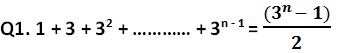Solution:

Let the given statement be P(n), i.e.,LHS = RHS

Thus P(k + 1) is true, whenever P (k) is true.

Hence, from the principle of mathematical induction, the statement P(n) is true for all natural numbers n.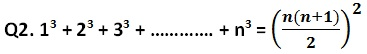Solution:

Let the given statement be P(n), i.e.,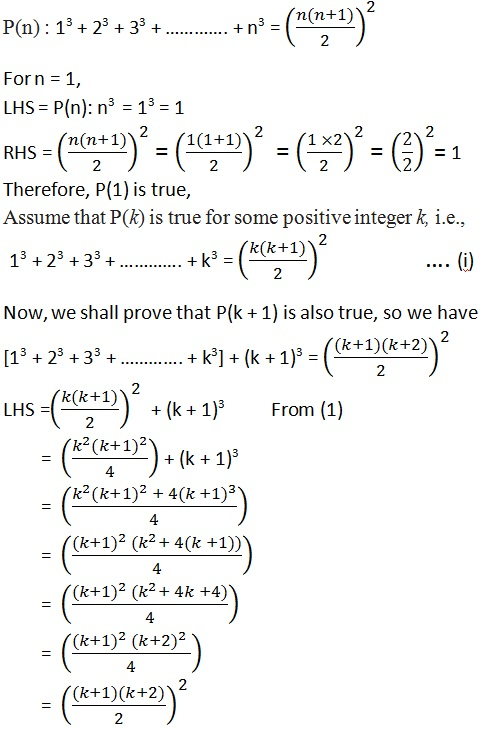LHS = RHS

Thus P(k + 1) is true, whenever P (k) is true.

Hence, from the principle of mathematical induction, the statement P(n) is true for all natural numbers n.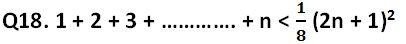Solution: Let the given statement be P(n), so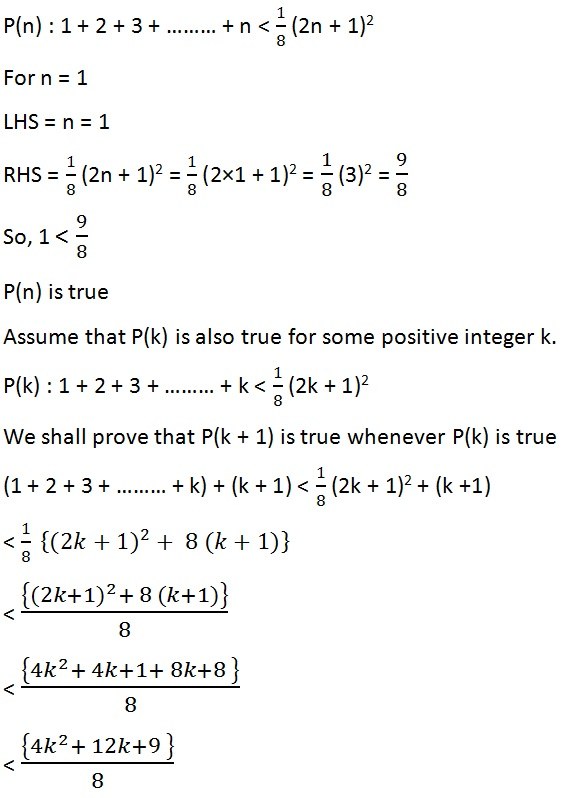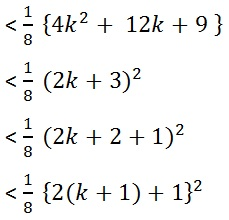Thus P(k + 1) is true, whenever P (k) is true.

Hence, from the principle of mathematical induction, the statement P(n) is true for all natural numbers n.

Q19. n (n + 1) (n + 5) is a multiple of 3.

Solution:

Let the given statement be P(n), so

P(n) : n (n + 1) (n + 5) is a multiple of 3.

For n = 1, so we have;

n (n + 1) (n + 5) = 1 × 2 × 6 = 12 = 3 × 4

P(n) is true for n = 1

Assume that P(k) is also true for some positive integer k.

k(k + 1) (k + 5)

= k3 + 6k2 + 5 k = 3m (say)   ……………….. (1)

Now, we shall prove that P(k + 1) is true whenever P(k) is true

Replacing k by k + 1

k + 1 (k + 2) (k + 6)

= (k + 1) (k2 + 8k + 12)

= k (k2 + 8k + 12) + 1(k2 + 8k + 12)

= k3 + 8k2 + 12k + k2 + 8k + 12

= k3 + 9k2 + 20k + 12

=( k3 + 6k2 + 5 k) + 3k2 + 15k + 12

= 3m + 3k2 + 15k + 12     from (1)

= 3(m + k2 + 5k + 4)

∴  k + 1 (k + 2) (k + 6) is multiple of 3

Thus P(k + 1) is true, whenever P (k) is true.

Hence, from the principle of mathematical induction, the statement P(n) is true for all natural numbers n ∈ N.

Q20.  102n - 1  + 1 is divisible by 11.

Solution:

Let the given statement be P(n), so

P(n) : 102n - 1  + 1 is divisible by 11.

For n = 1, so we have;

102n - 1  + 1 = 102×1 - 1 + 1 = 10 + 1 = 11

P(n) is true for n = 1

Assume that P(k) is also true for some positive integer k.

102k- 1  + 1  = 11m say

102k- 1  = 11m - 1      ……………… (1)

We shall prove that P(k + 1) is true whenever P(k) is true

replacing k by k + 1 we have

102k - 1  + 1

=  102k + 1  + 1

= 102k × 101 + 1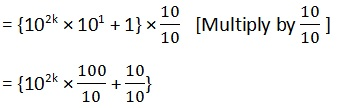= {102k - 1 × 100 + 1}

= {(11m - 1)× 100 + 1}     from equation (1)

= 1100m - 100+ 1

= 1100m - 99

= 11(100m - 9)

102n - 1  + 1 is divisible by 11

Thus P(k + 1) is true, whenever  P(k) is true.

Hence, from the principle of mathematical induction, the statement P(n) is true for all natural numbers n N.

Q21.  x2n y2n is divisible by x + y

Solution: Let the given statement be P(n), so

P(n) : x2n – y2n is divisible by x + y

Putting n = 1 we have,

x2n – y2n = x2 - y2 = (x + y) (x - y)

P(n) is true for n = 1

Assume that P(k) is also true for some positive integer k or

x2k – y2k is divisible by (x + y)

So, x2k – y2k = m( x + y)

Or  x2k = m( x + y) + y2k     …………. (1)

We shall prove that P(k + 1) is true whenever P(k) is true

replacing k by k + 1 we have

x2k + 2 – y2k + 2

= x2k . x2  – y2k .y2

Putting the value of x2k from (1)

= {m( x + y) + y2k} x2  – y2k .y2

= m( x + y) x2 + y2k. x2  – y2k .y2

= m( x + y) x2 + y2k (x2  – y2)

= m( x + y) x2 + y2k (x + y) ( x - y)

= ( x + y) [mx2 + y2k ( x - y)]

x2n – y2n is divisible by x + y

Thus P(k + 1) is true, whenever  P(k) is true.

Hence, from the principle of mathematical induction, the statement P(n) is true for all natural numbers n N.

Q22.  32n+2 – 8n – 9 is divisible by 8

Solution: Let the given statement be P(n), so

P(n) : 32n+2 – 8n – 9 is divisible by 8
Putting n =1

P(1) : 32×1+2 – 8 × 1 – 9 = 81 - 17 = 64 = 8 × 8

Which is divisible by 8

P(1) is true

Assume that P(k) is also true for some positive integer k

32k + 2 – 8k – 9

32k + 2 – 8k – 9 is divisible by 8
32k + 2 – 8k – 9 = 8m

Or 32k + 2 = 8m + 8k + 9     ……………. (1)

We shall prove that P(k + 1) is true whenever P(k) is true

replacing k by k + 1 we have

32k + 4 – 8k 8  – 9

= 32k + 4 – 8k 17

= 32k + 2 × 32 – 8k 17

= (8m + 8k + 9)× 9 – 8k 17

= 72m + 72k + 81 – 8k 17

= 72m + 64k + 64

= 8(9m + 8k + 8)

∴  32n+2 – 8n – 9 is divisible by 8

Thus P(k + 1) is true, whenever  P(k) is true.

Hence, from the principle of mathematical induction, the statement P(n) is true for all natural numbers n N.

Q23. 41n – 14n is a multiple of 27.

Solution: Let the given statement be P(n), so

P(n) : 41n – 14n is a multiple of 27
Putting n = 1

P(1): 41n – 14n = 41 – 14 = 27

P(1) is true

Assume that P(k) is also true for some positive integer k

41k – 14k = 27

41k = 27 + 14k   ………… (1)

We shall prove that P(k + 1) is true whenever P(k) is true

replacing k by k + 1 we have

41k + 1 – 14k + 1

=  41k . 41 – 14k . 14

=  (27 + 14k) 41 – 14k . 14

=  27 . 41 + 14k .41 – 14k . 14

=  27 . 41 + 14k (41 – 14 )

=  27 . 41 + 14k . 27

=  27 ( 41 + 14k )

41n – 14n is a multiple of 27
Thus P(k + 1) is true, whenever  P(k) is true.

Hence, from the principle of mathematical induction, the statement P(n) is true for all natural numbers n N.

Q24. (2n + 7) < (n + 3)2

Solution: Let the statement be p(n) so,

p(n) : (2n + 7) < (n + 3)2

=> p(1) :  (2 × 1 + 7) < (1 + 3)2

=> 9 < 42

=> 9 < 16

Therefore, p(1) is true so Assume that p(k) is also true for some integer k.

(2k + 7) < (k + 3)2  ......... (i)

Now we shall prove for p(k + 1)

2(k +1) + 7 < (k + 1 + 3)2

2k + 2 + 7 < (k + 4)2  ........ (ii)

We have from (i)

(2k + 7) < (k + 3)2

=> 2k + 7 + 2 < (k + 3)2 + 2

=> 2k + 7 + 2 < k2 + 6k + 9 + 2

=> 2k + 7 + 2 < k2​ + 6k + 9 + 2

=> 2k + 7 + 2 < k2​ + 6k + 11

Now, k2​ + 6k + 11 < (k + 4)2 from (ii)

=> 2k + 7 + 2 < k+ 6k + 11 < k2 + 8k + 16

=> 2k + 2 + 7 < k2 + 8k + 16

=> 2(k + 1) + 7 < (k + 4)2

=> 2(k + 1) + 7 < (k + 1 + 3)2

Thus P(+ 1) is true, whenever  P(k) is true.

Hence, from the principle of mathematical induction, the statement P(n) is true for all natural numbers n ∈ N.

##### Other Pages of this Chapter: 4. Principle Of Mathematical Induction

Important Study materials for classes 06, 07, 08,09,10, 11 and 12. Like CBSE Notes, Notes for Science, Notes for maths, Notes for Social Science, Notes for Accountancy, Notes for Economics, Notes for political Science, Noes for History, Notes For Bussiness Study, Physical Educations, Sample Papers, Test Papers, Mock Test Papers, Support Materials and Books.Mathematics Class - 11th

NCERT Maths book for CBSE Students.

books

## Study Materials List:

##### Solutions ⇒ Class 11th ⇒ Mathematics
1. Sets
2. Relations and Functions
3. Trigonometric Functions
4. Principle Of Mathematical Induction
5. Complex Numbers and Quadratic Equations
6. Linear Inequalities
7. Permutations and Combinations
8. Binomial Theorem
9. Sequences and Series
10. Straight Lines
11. Conic Sections
12. Introduction to Three Dimensional Geometry
13. Limits and Derivatives
14. Mathematical Reasoning
15. Statistics
16. Probability

New Books